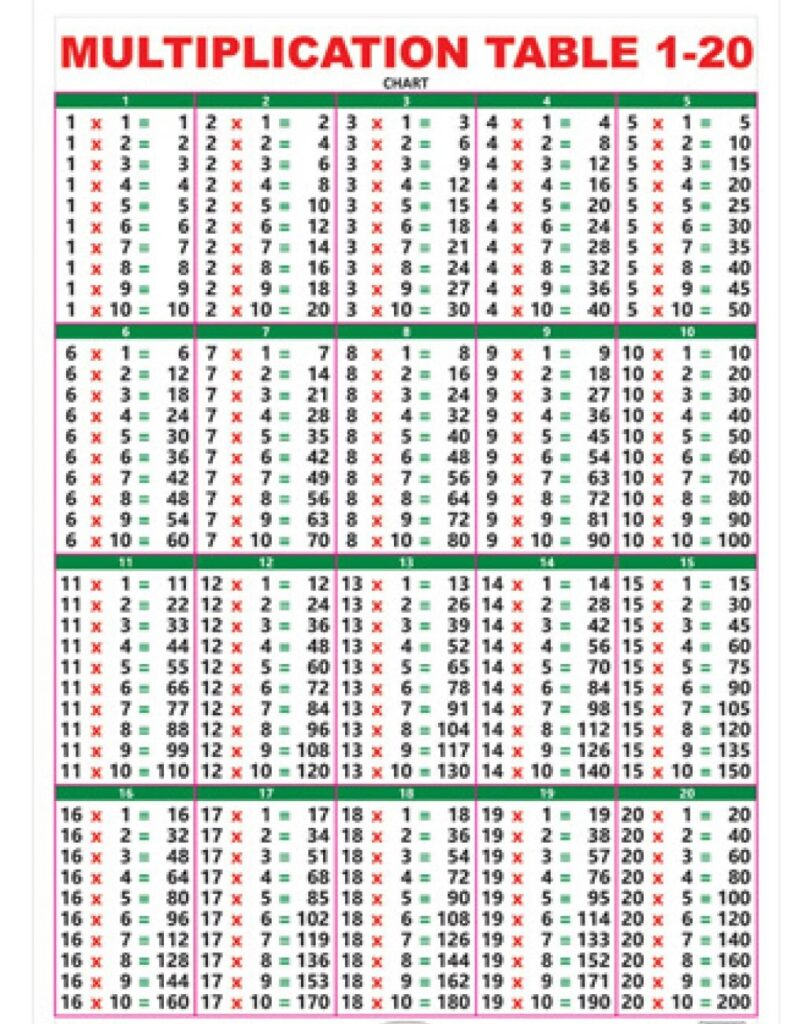# Tables 2 to 20

Tables 2 to 20 :- Table are required to do Multiplications, Divisions and other mathematical operations. Here on this page we have provided you Tables 2 to 20 in a very basic and eye catchy manner. Hope it will help you.

 Table of 11 × 1 = 11 × 2 = 21 × 3 = 31 × 4 = 41 × 5 = 51 × 6 = 61 × 7 = 71 × 8 = 81 × 9 = 91 × 10 = 10 Table of 22 ×‌ 1 = 22 ×‌ 2 = 42 × ‌3 = 62 × ‌4 = 82 × ‌5 = 102 × ‌6 = 122 × ‌7 = 142 × ‌8 = 162 × ‌9 = 182 × ‌10 = 20 Table of 33 × ‌1 = 33 × ‌2 = 63 × ‌3 = 93 × ‌4 = 123 × ‌5 = 153 × ‌6 = 183 × ‌7 = 213 × ‌8 = 243 × ‌9 = 273 × ‌10 = 30 Table of 44 × ‌1 = 44 × ‌2 = 84 × ‌3 = 124 × ‌4 = 164 × ‌5 = 204 × ‌6 = 244 × ‌7 = 284 × ‌8 = 324 × ‌9 = 364 × ‌10 = 40 Table of 55 × ‌1 = 55 × ‌2 = 105 × ‌3 = 155 × ‌4 = 205 × ‌5 = 255 × ‌6 = 305 × ‌7 = 355 × 8 = 405 × 9 = 455 × 10 = 50 Table of 66 × 1 = 66 × 2 = 126 × 3 = 186 × 4 = 246 × 5 = 306 × 6 = 366 × 7 = 426 × 8 = 486 × 9 = 546 × 10 = 60 Table of 77 × 1 = 77 × 2 = 147 × 3 = 217 × 4 = 287 × 5 = 357 × 6 = 427 × 7 = 497 × 8 = 567 × 9 = 637 × 10 = 70 Table of 88 × 1 = 88 × 2 = 168 × 3 = 248 × 4 = 328 × 5 = 408 × 6 = 488 × 7 = 568 × 8 = 648 × 9 = 728 × 10 = 80 Table of 99 × 1 = 99 × 2 = 189 × 3 = 279 × 4 = 369 × 5 = 459 × 6 = 549 × 7 = 639 × 8 = 729 × 9 = 819 × 10 = 90 Table of 1010 × 1 = 1010 × 2 = 2010 × 3 = 3010 × 4 = 4010 × 5 = 5010 × 6 = 6010 × 7 = 7010 × 8 = 8010 × 9 = 9010 × 10 = 100
 Table of 1111 ×‌‌ 1 = 1111 ×‌‌ 2 = 2211 ×‌‌ 3 = 3311 ×‌ 4 = 4411 ×‌ 5 = 5511 ×‌ 6 = 6611 ×‌ 7 = 7711 ×‌ 8 = 8811 ×‌ 9 = 9911 ×‌ 10 = 110 Table of 1212 ×‌ 1 = 1212 ×‌ 2 = 2412 ×‌ 3 = 3612 ×‌ 4 = 4812 ×‌ 5 = 6012 ×‌ 6 = 7212 ×‌ 7 = 8412 ×‌ 8 = 9612 ×‌ 9 = 10812 ×‌ 10 = 120 Table of 1313 ×‌ 1 = 1313 ×‌ 2 = 2613 ×‌ 3 = 3913 ×‌ 4 = 5213 ×‌ 5 = 6513 ×‌ 6 = 7813 ×‌ 7 = 9113 ×‌ 8 = 10413 ×‌ 9 = 11713 ×‌ 10 = 130 Table of 1414 ×‌ 1 = 1414 ×‌ 2 = 2814 ×‌ 3 = 4214 ×‌ 4 = 5614 ×‌ 5 = 7014 ×‌ 6 = 8414 ×‌ 7 = 9814 ×‌ 8 = 11214 ×‌ 9 = 12614 ×‌ 10 = 140 Table of 1515 ×‌ 1 = 1515 ×‌ 2 = 3015 ×‌ 3 = 4515 ×‌ 4 = 6015 ×‌ 5 = 7515 ×‌ 6 = 9015 ×‌ 7 = 10515 ×‌ 8 = 12015 ×‌ 9 = 13515 ×‌ 10 = 150 Table of 1616 ×‌ 1 = 1616 ×‌ 2 = 3216 ×‌ 3 = 4816 ×‌ 4 = 6416 ×‌ 5 = 8016 ×‌ 6 = 9616 ×‌ 7 = 11216 ×‌ 8 = 12816 ×‌ 9 = 14416 ×‌ 10 = 160 Table of 1717 ×‌ 1 = 1717 ×‌ 2 = 3417 ×‌ 3 = 5117 ×‌ 4 = 6817 ×‌ 5 = 8517 ×‌ 6 = 10217 ×‌ 7 = 11917 ×‌ 8 = 13617 ×‌ 9 = 15317 ×‌ 10 = 170 Table of 1818 ×‌ 1 = 1818 ×‌ 2 = 3618 ×‌ 3 = 5418 ×‌ 4 = 7218 ×‌ 5 = 9018 ×‌ 6 = 10818 ×‌ 7 = 12618 ×‌ 8 = 14418 ×‌ 9 = 16218 ×‌ 10 = 180 Table of 1919 ×‌ 1 = 19 19 ×‌ 2 = 3819 ×‌ 3 = 5719 ×‌ 4 = 7619 ×‌ 5 = 9519 ×‌ 6 = 11419 ×‌ 7 = 13319 ×‌ 8 = 15219 ×‌ 9 = 17119 ×‌ 10 = 190 Table of 2020 ×‌ 1 = 2020 ×‌ 2 = 4020 ×‌ 3 = 6020 ×‌ 4 = 8020 ×‌ 5 = 10020 ×‌ 6 = 12020 ×‌ 7 = 14020 ×‌ 8 = 16020 ×‌ 9 = 18020 ×‌ 10 = 200